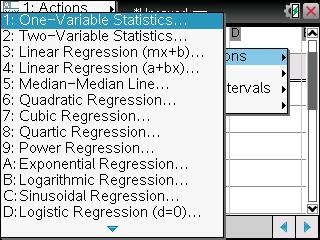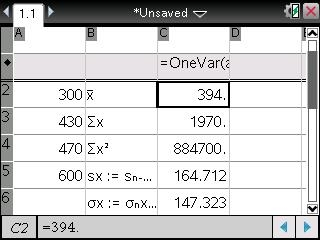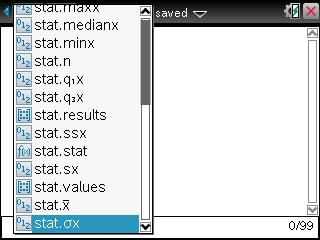# Knowledge Base

## Solution 31931: Calculating Variance Using the TI-Nspire™ Family Handhelds.

### How do I calculate variance using the TI-Nspire family handhelds?

The TI-Nspire family handhelds will calculate two types of standard deviation: the sample standard deviation and the population standard deviation. The population standard deviation, denoted by óx, divides the calculated values by n. The sample standard deviation, denoted by Sx, uses the value n-1 as the denominator.

The graphing calculators use the sample standard deviation Sx when calculating the variance (Sx2).

To find the variance using the population standard deviation, take the value of óx and raise it to the power of 2 (óx2).

Please Note: The óx variable will not have a value stored to it unless a 1-Var or 2-Var Stats analysis is performed first. Without a value stored to óx, calculating óx2 will result in an "UNDEFINED" error. For example: Using the data {170, 300, 430, 470, 600} find the variance.

1) Press [home].
2) Press 1: New Document.

Under Column A, enter the following data points:

4) Input 170 and press [enter].
5) Input 300 and press [enter].
6) Input 430 and press [enter].
7) Input 470 and press [enter].
8) Input 600 and press [enter].10) Press 4: Statistics.
11) Press 1: Stat Calculations.
12) Press 1: One-Variable Statistics.13) Input 1 for the Num of Lists and tab to 'OK' and press [enter].
14) Leave defaults set and tab to "OK' and press [enter].15) Press [ctrl] [+page].17) Press [var].
18) Scroll down to stat.óx and press [enter].19) Press [x2] and press [enter].Please Note: The variance( command will find the sample variance which is the sample standard deviation of x raised to the power of 2.

Please see the TI-Nspire CX, TI-Nspire CX CAS, TI-Nspire, and TI-Nspire CAS guidebooks for additional information.